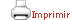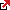Teaching plan for the course unit

 CloseGeneral information

Course unit name: Computational Complexity

Course unit code: 569077

Coordinator: Joost Johannes Joosten

Department: Faculty of Philosophy

Credits: 6

Single program: SEstimated learning time Total number of hours 150

 Face-to-face and/or online activities 56
 -  Lecture Face-to-face 28 -  Lecture with practical component Face-to-face 14 -  Problem-solving class Face-to-face 14
 Supervised project 40 (eight times five homework sessions)
 Independent learning 54

 Competences to be gained during study

Competences

Technical Competences of each Specialization

• CEE3.1 - Capability to identify computational barriers and to analyze the complexity of computational problems in different areas of science and technology as well as to represent high complexity problems in mathematical structures which can be treated effectively with algorithmic schemes.
• CEE3.3 - Capability to understand the computational requirements of problems from non-informatics disciplines and to make significant contributions in multidisciplinary teams that use computing.

Generic Technical Competences

Generic

• CG1 - Capability to apply the scientific method to study and analyse of phenomena and systems in any area of Computer Science, and in the conception, design and implementation of innovative and original solutions.
• CG3 - Capacity for mathematical modeling, calculation and experimental designing in technology and companies engineering centers, particularly in research and innovation in all areas of Computer Science.

Transversal Competences

Reasoning

• CTR6 - Capacity for critical, logical and mathematical reasoning. Capability to solve problems in their area of study. Capacity for abstraction: the capability to create and use models that reflect real situations. Capability to design and implement simple experiments, and analyze and interpret their results. Capacity for analysis, synthesis and evaluation.

Basic

• CB8 - Capability to communicate their conclusions, and the knowledge and rationale underpinning these, to both skilled and unskilled public in a clear and unambiguous way.
• CB9 - Possession of the learning skills that enable the students to continue studying in a way that will be mainly self-directed or autonomous.

 Learning objectives
 Referring to knowledge Understand different computational models and there interrelations. Understand the concept of (space, time) complexity classes (deterministic, non-deterministic, probabilistic). Understand Lower Bounds in Circuit Complexity and their importance. Understand the impact of computational complexity, both to theoretical computer science and to applied computer science.  Referring to abilities, skills Be able to identify inherent computational barriers. Be able to model computational processes and analyze the complexity of computational problems. Know how to use the main pointers in the literature and be able to place a paper in the literature landscape. Consolidate the previously defined competences and being able to apply them in various contexts both pure and applied.  Referring to attitudes, values and norms Lose fear of complex processes and acquire an attitude to start analyzing the most important (or understandable) parts of these processes. Acquire the norm to place scientific activities and contributions within the literature landscape with due references to the main contributors.

 Teaching blocks

1. Computational Models and Complexity Measures

*

1. Turing machine model. RAM model.
2. Boolean circuit model.
3. Time complexity.
4.  Space complexity.
5. Circuit size.
6. Circuit depth.
7. Time and space hierarchy theorems.

2. P, NP and NP-completeness

*

1. Polynomial time.
2. Reducibilities.
3. Non-deterministic algorithms and class NP.
4. Cook-Levin Theorem.
5. Many other NP-complete problems.

3. Polynomial-time Hierarchy and Alternations

*

1. Oracle reducibility.
2. NP and co-NP.
3. Levels of the hierarchy.
4. Quantifier alternations.
5. Complete problems.

4. Space Complexity

*

1. Polynomial space.
2. Unbounded alternations.
3. PSPACE-complete problems.
4. Savitch Theorem.
5. Immerman-Szcelepscenyi Theorem.
6. Logarithmic space.
7. NL-complete problems.

5. Randomized Computation

*

1. Bounded-error and zero-error probabilistic polynomial time.
2. Error-reduction.
3. Randomized reductions.
4. Valiant-Vazirani reduction to Unique SAT.

6. Counting and Enumeration

*

1. Some examples: graph reliability, counting matchings and the permanent, partition functions.
2. Counting computation paths in non-deterministic machines.
3. Valiant’s Theorem.
4. Random self-reducibility of the permanent.

7. Probabilistic Proofs

*

1. Interaction and randomness in proofs.
2. Probabilistic proofs for graph non-isomorphism.
3. Probabilistic proofs for #P and Shamir’s Theorem: IP = PSPACE.

8. Circuit Lower Bounds

*

1. Monotone circuits.
2. Lower bounds for clique and perfect matching.
3. Bounded-depth circuits.
5. Approximation by polynomials.

 Teaching methods and general organization

 Blackboard lectures for theory classes and discussion sessions for the problem classes. The theory classes will follow the main textbook for the class [Arora and Barak] rather closely. Since we plan to cover more topics than is possible in the given time, students will be required to read the details in the textbook as homework (a draft of the book is available on-line for free). The aim of the discussion sessions is to solve some problems from that book and to discuss the reading material.

 Official assessment of learning outcomes

 Students will be required to submit 5 problem/discussion sheets. Each will be given a grade in [0,1] (P1,...,P5).There will be a final exam graded in [0,10] (E).The final grade of the course will be MAX(P1+P2+P3+P4+P5+E/2, E).The problem/discussion sheets will consist of problems from the main textbook [Arora-Barak] and/or multiple choice questions that test if the student understood the material from the theory class (also covered in the main textbook).   Examination-based assessment There will be a final exam graded in [0,10]